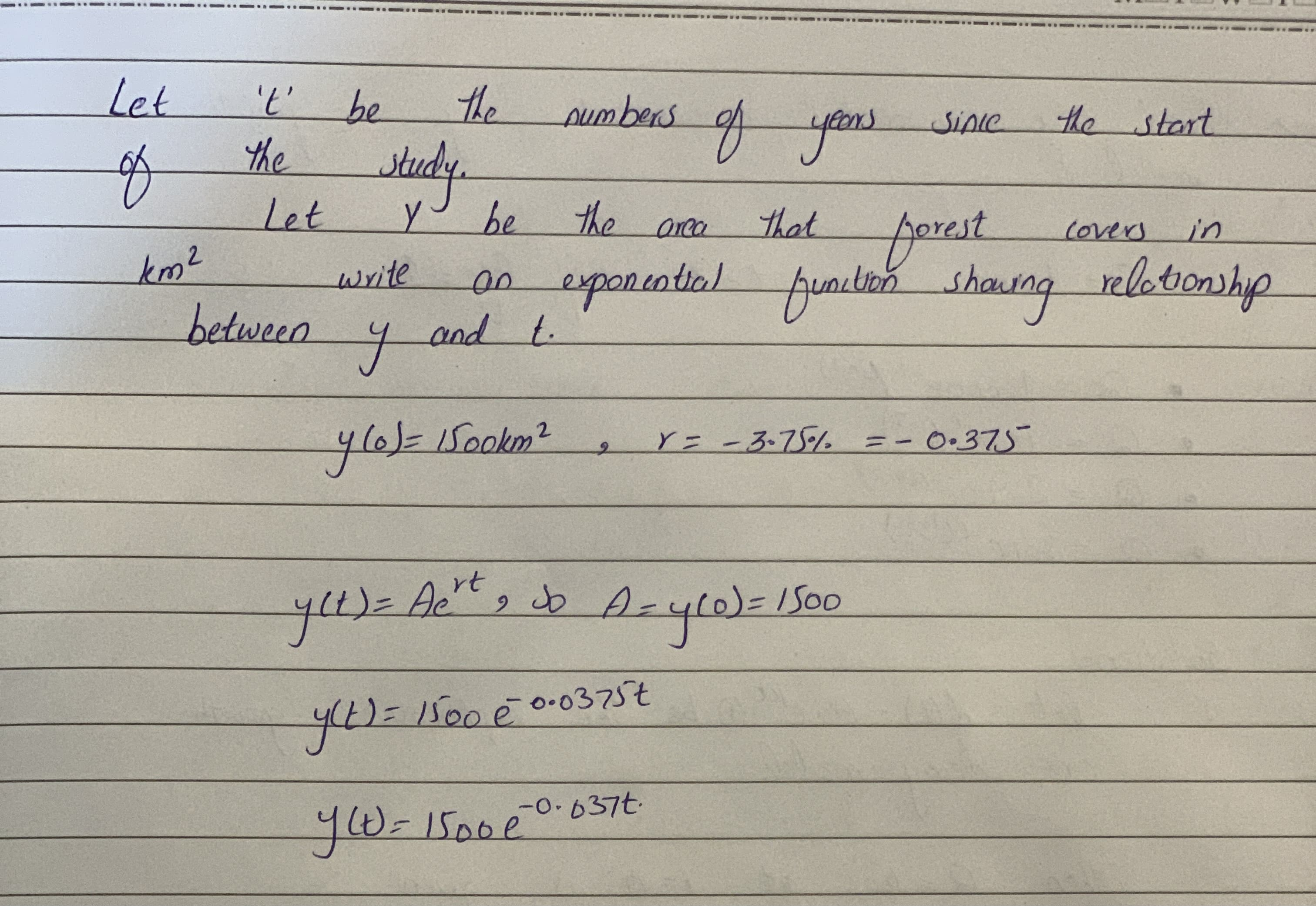# At the beginning of an environmental study, ad forest covered an area of 1500 km^2.Since then, this area has decreased by 3.75% each year.Let t be theDaniaal Sanchez 2021-02-12 Answered
At the beginning of an environmental study, ad forest covered an area of $1500k{m}^{2}$.Since then, this area has decreased by 3.75% each year.Let t be the number of years since the start of the study.Let y be the area that the forest covers in $k{m}^{2}$.
Write an exponential function showing relationship between y and t.
You can still ask an expert for help

## Want to know more about Exponential growth and decay?

• Questions are typically answered in as fast as 30 minutes

Solve your problem for the price of one coffee

• Math expert for every subject
• Pay only if we can solve itrogreenhoxa8................